# Test: Asymptotic Worst Case Time & Space Complexity- 2

## 20 Questions MCQ Test GATE Computer Science Engineering(CSE) 2023 Mock Test Series | Test: Asymptotic Worst Case Time & Space Complexity- 2

Description
Attempt Test: Asymptotic Worst Case Time & Space Complexity- 2 | 20 questions in 60 minutes | Mock test for Computer Science Engineering (CSE) preparation | Free important questions MCQ to study GATE Computer Science Engineering(CSE) 2023 Mock Test Series for Computer Science Engineering (CSE) Exam | Download free PDF with solutions
QUESTION: 1

### A list of n string, each of length n, is sorted into lexicographic order using the merge-sort algorithm. The worst case running time of this computation is

Solution:

The recurrence tree for merge sort will have height Log(n). And O(n^2) work will be done at each level of the recurrence tree (Each level involves n comparisons and a comparison takes O(n) time in worst case). So time complexity of this Merge Sort will be [Tex]O (n^2 log n) [/Tex].

QUESTION: 2

### In quick sort, for sorting n elements, the (n/4)th smallest element is selected as pivot using an O(n) time algorithm. Q. What is the worst case time complexity of the quick sort?

Solution:

The recursion expression becomes: T(n) = T(n/4) + T(3n/4) + cn After solving the above recursion, we get  θ(nLogn).

QUESTION: 3

### Consider the Quicksort algorithm. Suppose there is a procedure for finding a pivot element which splits the list into two sub-lists each of which contains at least one-fifth of the elements. Let T(n) be the number of comparisons required to sort n elements. Then

Solution:

For the case where n/5 elements are in one subset, T(n/5) comparisons are needed for the first subset with n/5 elements, T(4n/5) is for the rest 4n/5 elements, and n is for finding the pivot. If there are more than n/5 elements in one set then other set will have less than 4n/5 elements and time complexity will be less than T(n/5) + T(4n/5) + n because recursion tree will be more balanced.

QUESTION: 4

Consider the following functions:

f(n) = 2^n
g(n) = n!
h(n) = n^logn

Q. Which of the following statements about the asymptotic behavior of f(n), g(n), and h(n) is true?

Solution:

According to order of growth: h(n) < f(n) < g(n) (g(n) is asymptotically greater than f(n) and f(n) is asymptotically greater than h(n) ) We can easily see above order by taking logs of the given 3 functions

lognlogn < n < log(n!) (logs of the given f(n), g(n) and h(n)).

Note that log(n!) =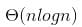QUESTION: 5

In the following C function, let n >= m.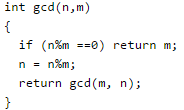Q. How many recursive calls are made by this function?

Solution:

Above code is implementation of the Euclidean algorithm for finding Greatest Common Divisor (GCD).

QUESTION: 6

Which of the following sorting algorithms has the lowest worst-case complexity?

Solution:

Worst case complexities for the above sorting algorithms are as follows: Merge Sort — nLogn Bubble Sort — n^2 Quick Sort — n^2 Selection Sort — n^2

QUESTION: 7

Consider the following functions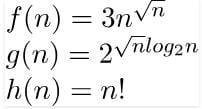Solution:

g(n) =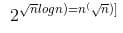f(n) and g(n) are of same asymptotic order and following statements are true. f(n) = O(g(n)) g(n) = O(f(n)). (a) and (b) are false because n! is of asymptotically higher order than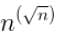.

QUESTION: 8

Consider the following three claims I (n + k)^m = θ(n^m), where k and m are constants II 2^(n + 1) = 0(2^n) III 2^(2n + 1) = 0(2^n) Which of these claims are correct?

Solution:

(I) (n+m)^k = n^k + c1*n^(k-1) + ... k^m = θ(n^k)
(II) 2^(n+1) = 2*2^n = θ(2^n)

QUESTION: 9

Let s be a sorted array of n integers. Let t(n) denote the time taken for the most efficient algorithm to determined if there are two elements with sum less than 1000 in s. which of the following statements is true?

Solution:

Let array be sorted in ascending order, if sum of first two elements is less than 1000 then there are  two elements with sum less than 1000 otherwise not. For array sorted in descending order we need to check last two elements. For an array data structure, number of operations are fixed in both the cases and not dependent on n, complexity is O(1)

QUESTION: 10

Consider the following function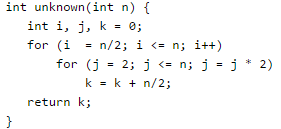Q. What is the returned value of the above function?

Solution:

The outer loop runs n/2 or θ times. The inner loop runs θ(Logn) times (Note that j is multiplied by 2 in every iteration). So the statement "k = k + n/2;" runs θ(nLogn) times. The statement increases value of k by n/2. So the value of k becomes n/2* θ(nLogn) which is θ(n^2Logn)

QUESTION: 11

The number of elements that can be sorted in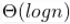time using heap sort is

Solution:

Time complexity of Heap Sort is θ(mLogm) for m input elements. For m =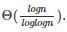, the value of θ(m * Logm) will be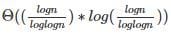which will be [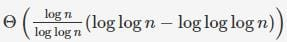which is θ(Log n)

QUESTION: 12

Consider the following two functions. What are time complexities of the functions?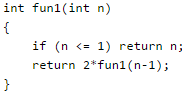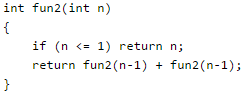Solution:

Time complexity of fun1() can be written as T(n) = T(n-1) + C which is O(n) Time complexity of fun2() can be written as T(n) = 2T(n-1) + C which is O(2^n)

QUESTION: 13

In quick sort, for sorting n elements, the (n/4)th smallest element is selected as pivot using an O(n) time algorithm. What is the worst case time complexity of the quick sort?

Solution:

Answer(B) The recursion expression becomes: T(n) = T(n/4) + T(3n/4) + cn After solving the above recursion, we get heta(nLogn).

QUESTION: 14

Consider the Quicksort algorithm. Suppose there is a procedure for finding a pivot element which splits the list into two sub-lists each of which contains at least one-fifth of the elements. Let T(n) be the number of comparisons required to sort n elements. Then

Solution:

For the case where n/5 elements are in one subset, T(n/5) comparisons are needed for the first subset with n/5 elements, T(4n/5) is for the rest 4n/5 elements, and n is for finding the pivot. If there are more than n/5 elements in one set then other set will have less than 4n/5 elements and time complexity will be less than T(n/5) + T(4n/5) + n because recursion tree will be more balanced.

QUESTION: 15

Consider the following segment of C-code: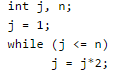Q. The number of comparisons made in the execution of the loop for any n > 0 is: Base of Log is 2 in all options.

Solution:

We can see it by taking few examples like n = 1, n = 3, etc.

For example, for n=5 we have the following (4) comparisons: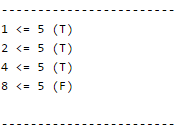CEIL(log_2 n)+2 = CEIL(log_2 5) + 2 = CEIL(2.3) + 2 = 3 + 2 = 5

QUESTION: 16

Consider the following C-program fragment in which i, j and n are integer variables.

for (i = n, j = 0; i >0; i /= 2, j += i);

Q. Let val(j) denote the value stored in the variable j after termination of the for loop. Which one of the following is true?

Solution:

The variable j is initially 0 and value of j is sum of values of i. i is initialized as n and is reduced to half in each iteration. j = n/2 + n/4 + n/8 + .. + 1 = Θ(n) Note the semicolon after the for loop, so there is nothing in the body.

QUESTION: 17

The minimum number of comparisons required to find the minimum and the maximum of 100 numbers is _________________.

Solution:

To find minimum and maximum element out of n numbers, we need to have at least (3n/2-2) comparisons.

QUESTION: 18

Consider the following pseudo code. What is the total number of multiplications to be performed?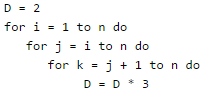Solution:
QUESTION: 19

You have an array of n elements. Suppose you implement quicksort by always choosing the central element of the array as the pivot. Then the tightest upper bound for the worst case performance is

Solution:

The central element may always be an extreme element, therefore time complexity in worst case becomes O(n2)

QUESTION: 20

Consider the following C-function: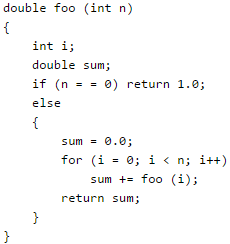Q. The space complexity of the above function is:

Solution:

Note that the function foo() is recursive. Space complexity is O(n) as there can be at most O(n) active functions (function call frames) at a time.Use Code STAYHOME200 and get INR 200 additional OFF Use Coupon Code